# 堆算法

## 什么是堆

（heap），是一类特殊的数据结构的统称。它通常被看作一棵树的数组对象。在队列中，调度程序反复提取队列中的第一个作业并运行，因为实际情况中某些时间较短的任务却可能需要等待很长时间才能开始执行，或者某些不短小、但很重要的作业，同样应当拥有优先权。而堆就是为了解决此类问题而设计的数据结构。

PARENT(i)
1   return 小于或等于i/2的最大整数

LEFT-CHILD(i)
1   return 2i

RIGHT-CHILD(i)
1   return 2i+1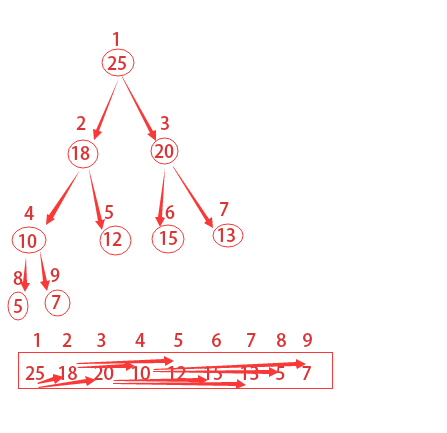## 通过MAX-HEAPIFY维护最大堆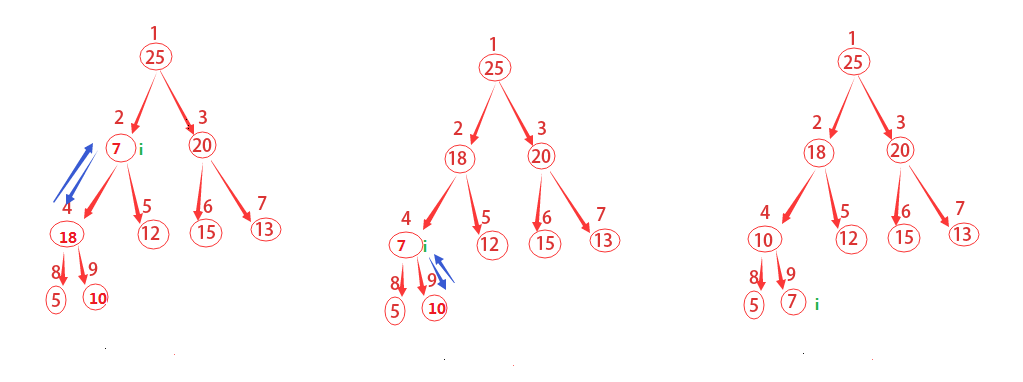MAX-HEAPIFY(A,i)
1   l=LEFT-CHILD(i)
2   r=RIGHT-CHILD(i)
3   if l<=A.heap-size and A[l]>A[i]
4       largest=l
5   else
6       largest=i
7   if r<=A.heap-size and A[r]>A[largest]
8       largest=r
9   if largest != i
10      exchange A[i] with A[largest]
11      MAX-HEAPIFY(A,largest)      

T(n)T(2n/3)+Θ(1)$T(n)\leq T(2n/3)+\Theta(1)$

T(n)=O(lgn)$T(n)=O(lgn)$

## 通过BUILD-MAX-HEAP构建最大堆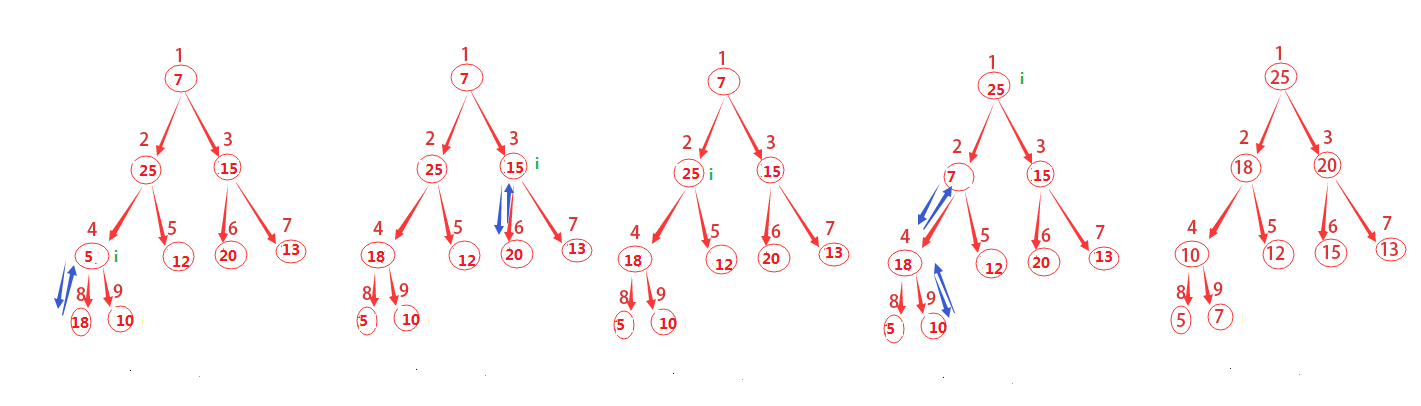BUILD-MAX-HEAP(A)
1   A.heap-size=A.length
2   for i=小于或等于A.length/2的最大整数 downto 1
3       MAX-HEAPIFY(A,i)

## 通过HEAPSORT进行堆排序算法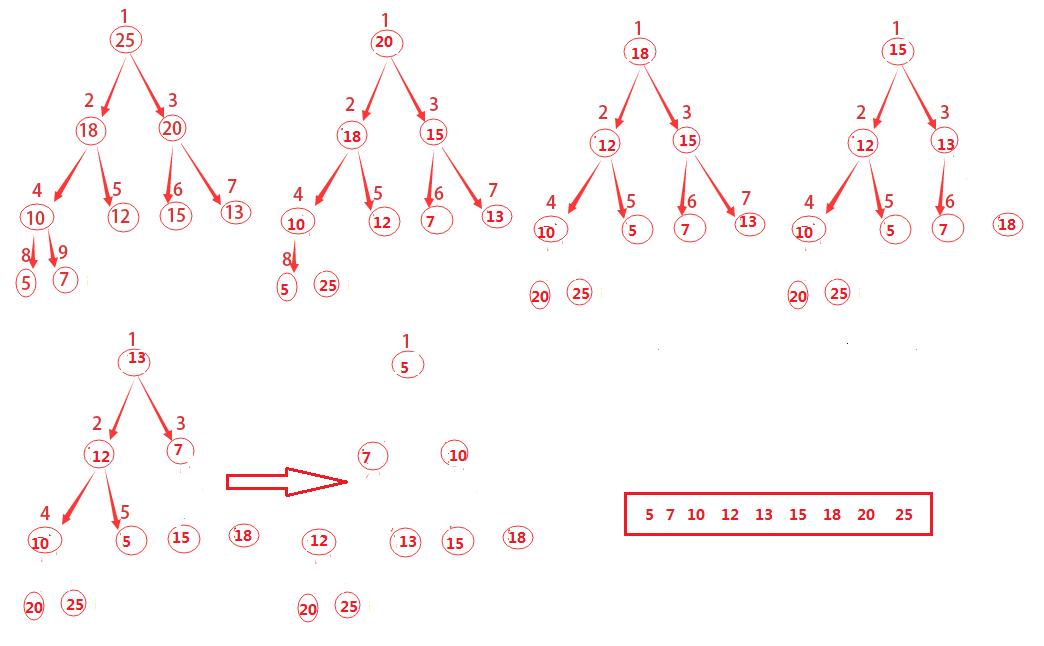1）将A$A$A[i]$A[i]$互换，i$i$A.length$A.length$一直递减到2

2）不断调用MAX-HEAPIFY(A,1)对剩余的整个堆进行重新构建

HEAPSORT(A)
1   BUILD-MAX-HEAP(A)
2   for i=A.length downto 2
3       exchange A with A[i]
4       A.heap-size=A.heap-size-1
5       MAX-HEAPIFY(A,1)

## 优先队列

MAXIMUM(S)$MAXIMUM(S)$：返回S$S$中有着最大键值的元素。
EXTRACTMAX(S)$EXTRACT-MAX(S)$：去掉并返回S$S$中的具有最大键字的元素。
INCREASEKEY(S,x,a)$INCREASE-KEY(S,x,a)$：将元素x$x$的关键字值增加到a$a$，这里假设a$a$的值不小于x$x$的原关键字值。
INSERT(S,x)$INSERT(S,x)$：将元素x$x$插入集合S$S$中，这一操作等价于S=S{x}$S=S\cup \{x\}$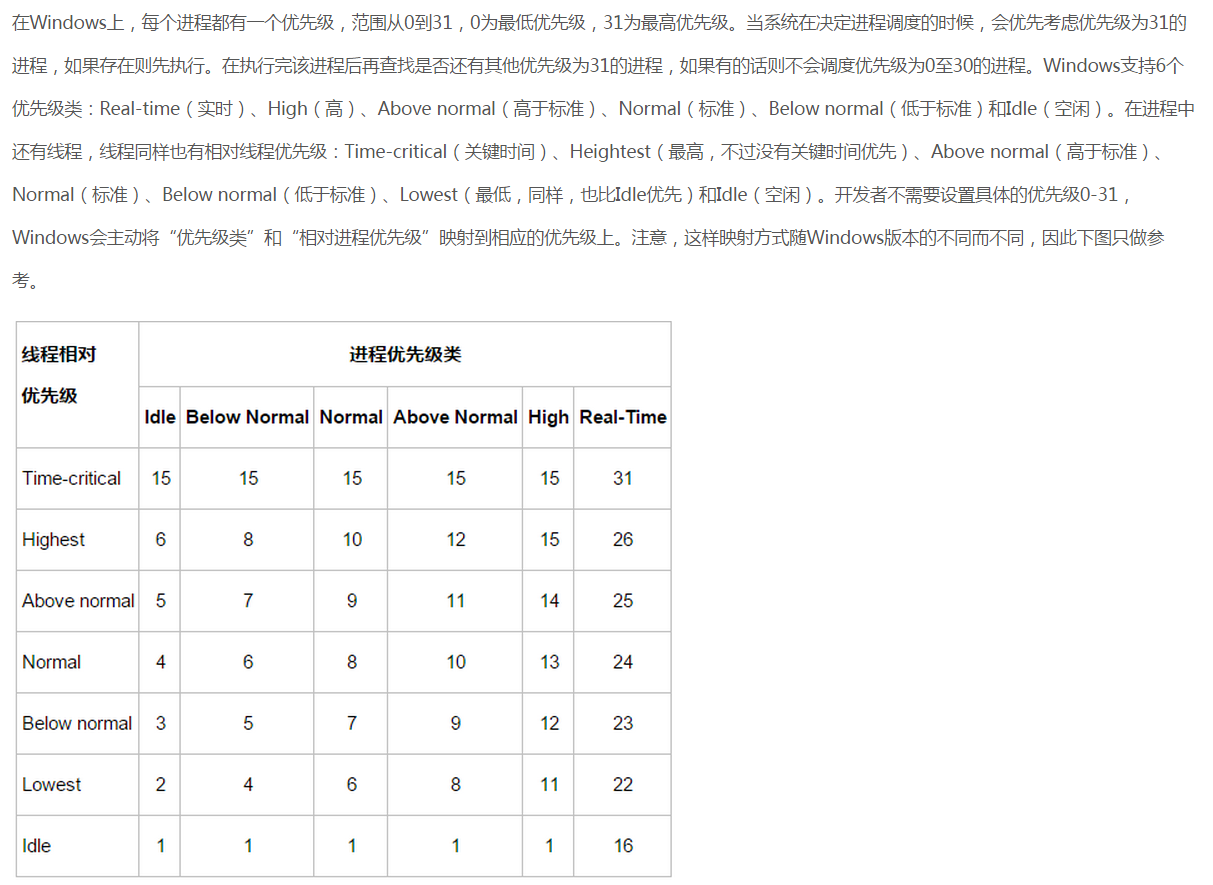HEAP-MAXIMUM(A)
1   return A

HEAP-EXTRACT-MAX(A)
1   if A.heap-size < 1
2       error "堆下溢"
3   max=A
4   A=A[A.heap-size]
5   A.heap-size=A.heap-size-1
6   MAX-HEAPIFY(A,1)
7   return max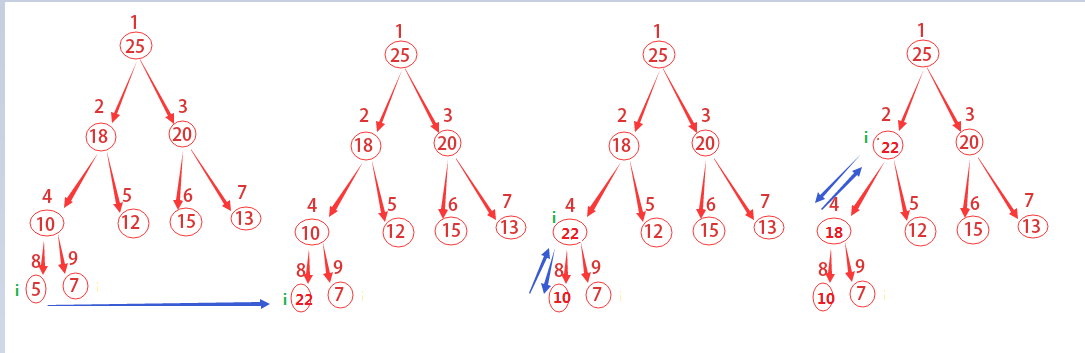HEAP-INCREASE-KEY(A,i,key)
1   if key < A[i]
2       error "新关键字值比当前关键字值更小"
3   A[i]=key
4   while i>1 and A[PARENT(i)] < A[i]
5       exchange A[i] with A[PARENT(I)]
6       i=PARENT(i)

MAX-HEAP-INSERT(A,key)
1   A.heap-size=A.heap-sieze+1
2   A[A.heap-size]=-10000
3   HEAP-INCREASE-KEY(A,A.hep-size,key)

# 辨析栈与队列

## 栈和队列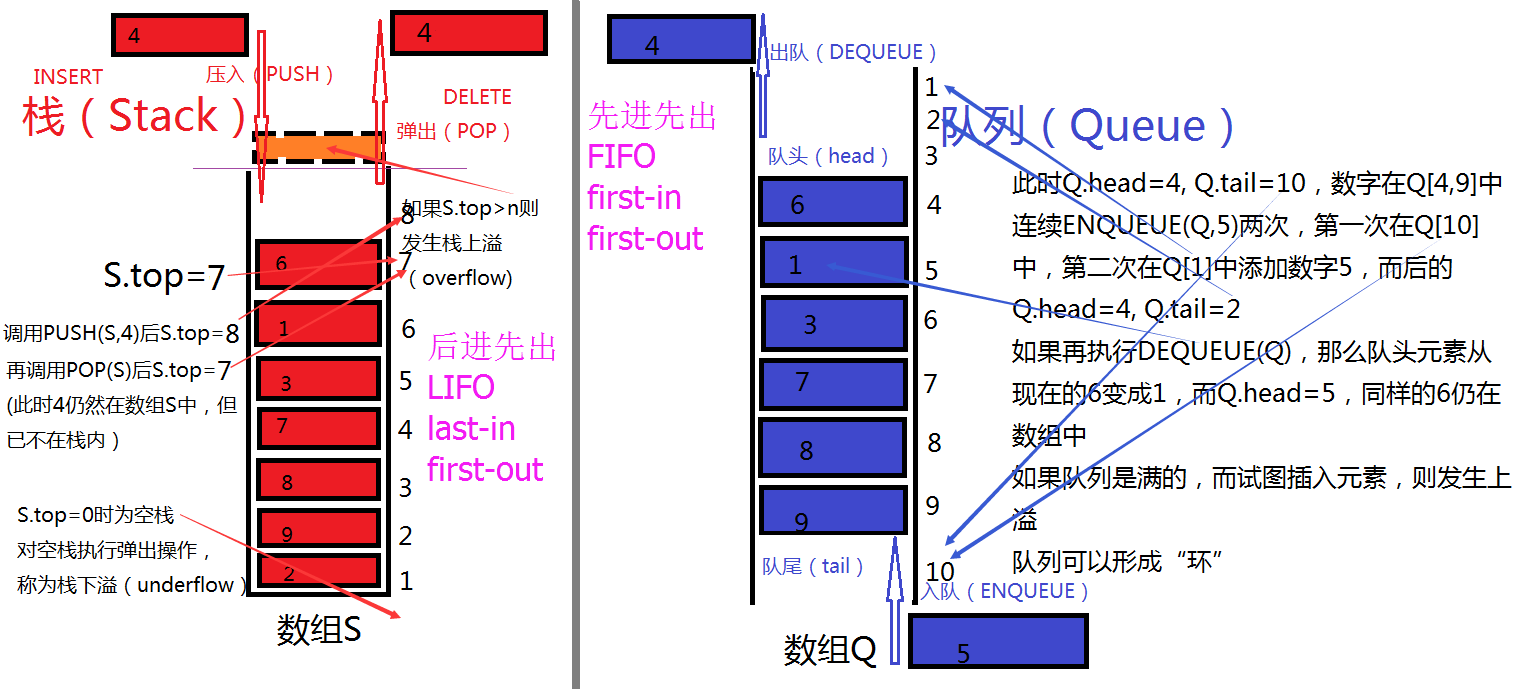STACK-EMPTY(S)
1   if S.top==0
2       return TRUE
3   else
4       return FLASE
PUSH(S,k)
1   S.top=S.top+1
2   S[S.top]=x
POP(S)
1   if STACK-EMPTY(S)
2       error "underflow"
3   else
4       S.top=S.top-1
5   return S[S.top+1]

ENQUEUE(Q,x)
1   Q[Q.tail]=x
2   if Q.tail==Q.length
3       Q.tail=1
4   else
5       Q.tail=Q.tail+1
DEQUEUE(Q)
4   else
6   return x

# 三种链表及其哨兵

## 三种链表的介绍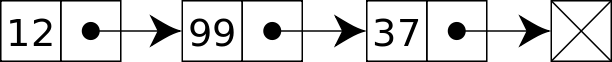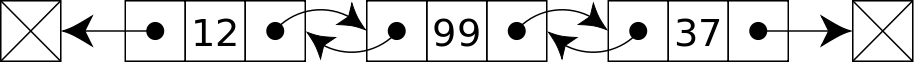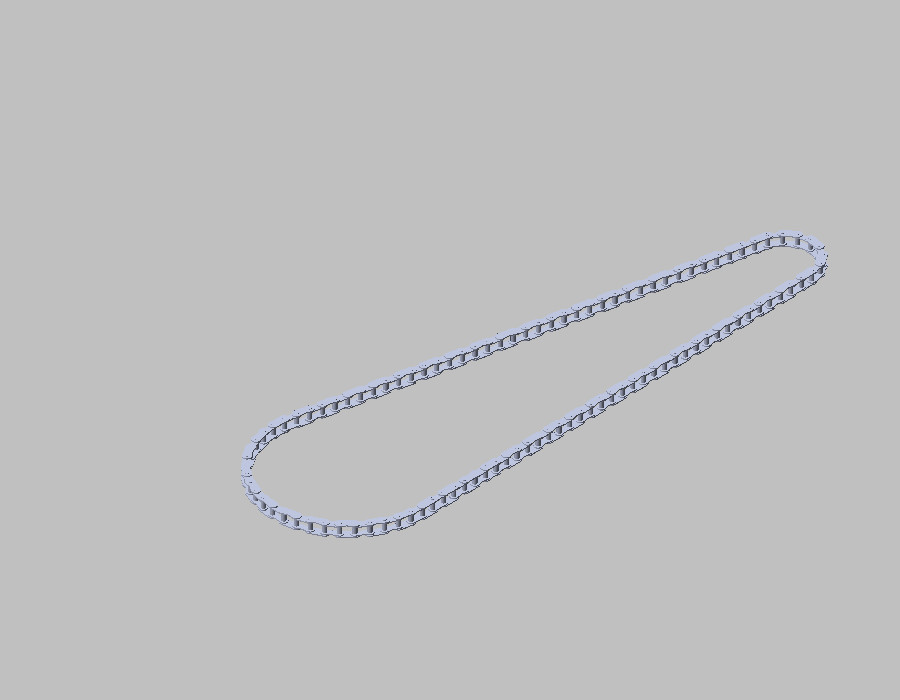## 链表的搜索、插入、删除

LIST-SEARCH(L,k)
2   while x!=NIL and x.key!=k
3       x=x.next
4   return x

LIST-INSERT(L,x)
5   x.prev=NIL

LIST-DELETE(L,x)
1   if x.prev!=NIL
2       x.prev.next=x.next
4   if x.next!=NIL
5       x.next.prev=x.prev

## 哨兵1）增加操作的速度
2）降低算法的复杂性和代码的大小
3）增加数据结构的鲁棒性

LIST-DELETE'(L,x)
1   x.prev.next=x.next
2   x.next.prev=x.prev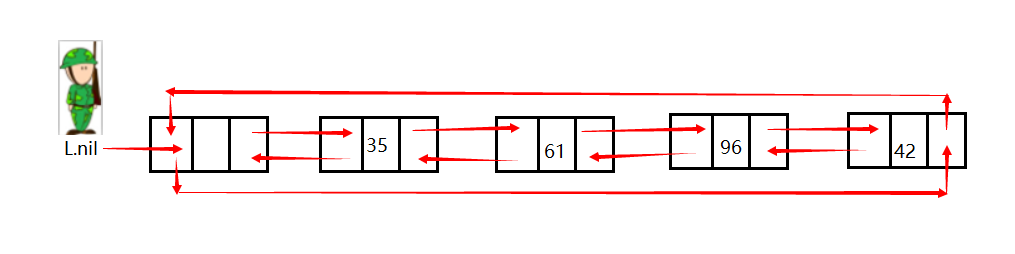L.nil就是守卫链表疆土的哨兵，那么L.nil.prev就自然的指向表尾了，相应的L.nil.prev指向表头。

LIST-SEARCH'(L,k)
1   x=L.nil.next
2   while x!=L.nil and x.key!=k
3       x=x.next
4   return x

LIST-INSERT'(L,x)
1   x.next=L.nil.next
2   L.nil.next.prev=x
3   L.nil.next=x
4   x.prev=L.nil